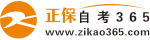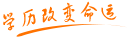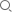#24小时客服：4008135555/010-82335555# 自考“C语言程序设计”模拟试题十三答案

2007年05月29日    来源：   字体：   打印

一、单项选择题：

1.D    2.B    3.D    4.B    5.B    6.B    7.A    8.B    9.B   10.C

11.A   12.C   13.B   14.C   15.A   16.D   17.B   18.C   19.A  20.B

二、填空题：

1.字母、数字和下划线

2.双引号

3.26

4.5

5.三（3）

6.1，20

7.1

8.顺序结构、选择结构、循环结构

9.类型不同

10.存储在外部介质上的相关数据集合。

三、将下列程序补充完整。

1.（1）（（a+b<c）||（b+c<a）||（a+c<b））（2）sqrt（t*（t-a）*（t-b）*（t-c））

2.（3）“%d%d”，&n1，&n2 （4） j=n1；n1=n2；n2=j；（5）j%3==0

3.（6）（k=2；k<=sqrt（x）；k++） （7） j=a；a=b；b=j；（8）prime（j）

4.（9）scanf（“%s”，&n）（10）else if （x==0）

5.（11）ch==`Q`||==`q`（12）ch>=`A`&&ch<=`Z`||ch>=`a`&&ch<=`z`

四、按格式写出程序运行结果

1.a10=1，a8=1，a16=1

c10=65，c8=101，c16=41，cc=A

d10=98，dc=b

2.8   5   2

k=4   y=0

3.m=82，j=7

4.ABCD

BCD

CD

D

五、按题目要求写出可运行程序

1.#include “stdio.h”

main（）

{ int term；

int i，max，min；

printf（“请输入10个整数：”）；

for（i=0；i<10；i++）

scanf（“%d”，&term[i]）；

（max=term；

min=term；

for（i=0；i<10；i++）

{if（term[i]<min）min=term[i]；

if（term[i]>max）max=term[i]；}

printf（“max=%d，min=%d”，max，min）；}

2. main（）

int j，k，x；

int a[]={9，7，5，3，1，2，4，6，8}；

for（j=0；j<3；j++）

for（k=j+1，k<3；k++）

{x=a[j][k]；a[j][k]=a[k][j]；a[k][j]=x；}

for（j=0；j<3；j++）

{for（k=0，k<3；k++）

printf（“%4d”，a[j][k]）；}}

3.#include “stdio.h”

int gcd（int m，int，n）

{if（n==0）return（m）；

else return（gcd（n，m%n））；

int tim（int m，int，n）

return（m*n/gcd（m，n））；}

main（）

{int m，n，g，t；

scanf（“%d，%d”，&m，&n）；

g=gcd（m，n）；t=tim（m，n）；

printf（“gcd（m，n）=%d\n”，g）；

printf（“tim（m，n）=%d\n”，t）；}

• 热门课程
• 报名咨询

• 相关问答
• 热门问答

• 购物车
• APP下载扫一扫，立即下载

安卓版本：

苹果版本：

开发者：北京正保自考教育科技有限公司

应用涉及权限：查看权限>>

APP隐私策略：查看政策>>

• 公众号扫一扫，立即关注

• 微信群进群领试题/资料